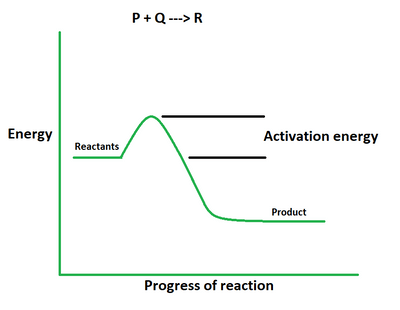GFG App
Open AppBrowser
Continue

# Activation Energy Formula

Activation energy of a chemical reaction is defined as the least amount of energy necessary to initiate the reaction. It can be interpreted as the differential in energy content between molecules and atoms that causes it to be in an activation or transition-state configuration while the associated atoms and molecules stay in their initial configuration. It is known that to initiate a reaction, molecules must collide with other molecules and exchange kinetic energy or velocity. There will be no response if the collision does not occur or if the molecules do not have enough kinetic energy. This forms the basis for the concept of activation energy. Its standard unit of measurement is kilojoules per mole (kJ/mol).Formula

Ea = 2.303 R (log k2/k1) [T1T2 / (T2 – T1)]

where,

Ea is the activation energy of the reaction,

R is the ideal gas constant with the value of 8.3145 J/K mol,

k1,k2 are the rates of reaction constant at initial and final temperature,

T1 is the initial temperature,

T2 is the final temperature.

This is also known as the Arrhenius equation.

Derivation

Suppose a reaction takes place between two reactants P and Q to give a product R. The completion of a reaction is ensured and denoted by its rate constant.

We know the formula for rate constant is given by,

k = Ae−EaRT

Taking log on both sides we get,

ln k = −EaRT + ln A

For initial temperature T1 and rate of constant k1, the equation is written as,

ln k1 = −Ea/RT1 + ln A   …….. (1)

For final temperature T2 and rate of constant k2, the equation is written as,

ln k2 = −Ea/RT2 + ln A   …….. (2)

Subtracting (2) from (1) we get,

ln k2 – ln k1 = −Ea/RT2 + ln A − (− Ea/RT1 + ln A)

ln (k2/k1) = EaR (1/T1 − 1/T2)

2.303 log (k2/k1) = EaR (1/T1 − 1/T2)

Ea = 2.303 R (log k2/k1) [T1T2 / (T2 – T1)]

This derives the formula for activation energy.

### Sample Problems

Problem 1. Calculate the activation energy of a reaction if its rate doubles when there is a temperature change from 200K to 400K.

Solution:

We have,

k2 = 2k1,

T1 = 200

T2 = 400

Using the formula we get,

Ea = 2.303 R (log k2/k1) [T1T2 / (T2 – T1)]

= 2.303 (8.3145) (log 2) (80000/200)

= 461090.907/200

= 2305.45 KJ/mol

Problem 2. Calculate the activation energy of a reaction if its rate triples when there is a temperature change from 100K to 300K.

Solution:

We have,

k2 = 3k1,

T1 = 100

T2 = 300

Using the formula we get,

Ea = 2.303 R (log k2/k1) [T1T2 / (T2 – T1)]

= 2.303 (8.3145) (log 3) (30000/200)

= 274012.079/200

= 1370.060 KJ/mol

Problem 3. Calculate the activation energy of a reaction if its rate quadruples when there is a temperature change from 150K to 400K.

Solution:

We have,

k2 = 4k1,

T1 = 150

T2 = 400

Using the formula we get,

Ea = 2.303 (8.3145) (log 4) (60000/250)

= 691636.36/250

= 2766.55 KJ/mol

Problem 4. Calculate the activation energy of a reaction if its rate becomes five times when there is a temperature change from 300K to 600K.

Solution:

We have,

k2 = 5k1,

T1 = 300

T2 = 600

Using the formula we get,

Ea = 2.303 (8.3145) (log 5) (180000/300)

= 2405791.59/300

= 8019.30 KJ/mol

Problem 5. Calculate the change in the rate of reaction if the activation energy of a reaction is 500 KJ/mol when there is a temperature change from 120K to 360K.

Solution:

We have,

Ea = 500

T1 = 120

T2 = 360

Using the formula we get,

=> 3000 = 2.303 (log k2/k1) [43200/240]

=> log (k2/k1) = (500/2.303) (240/43200)

=> log (k2/k1) = 7.23

=> k2/k1 = 16

=> k2 = 16 k1

Problem 6. Calculate the change in the rate of reaction if the activation energy of a reaction is 200 KJ/mol when there is a temperature change from 50K to 100K.

Solution:

We have,

Ea = 200

T1 = 50

T2 = 100

Using the formula we get,

=> 200 = 2.303 (log k2/k1) [5000/50]

=> log (k2/k1) = (200/2.303) (50/5000)

=> log (k2/k1) = 0.86

=> k2/k1 = 7.37

=> k2 = 7.37 k1

Problem 7. Calculate the change in the rate of reaction if the activation energy of a reaction is 450 KJ/mol when there is a temperature change from 100K to 200K.

Solution:

We have,

Ea = 450

T1 = 100

T2 = 200

Using the formula we get,

=> 450 = 2.303 (log k2/k1) [20000/100]

=> log (k2/k1) = (450/2.303) (100/20000)

=> log (k2/k1) = 0.97

=> k2/k1 = 9.33

=> k2 = 9.33 k1

My Personal Notes arrow_drop_up